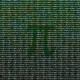# Arithmetic GasMathematical GardenPrime numbers can be considered as the building blocks of natural numbers. For example, 12 can be factored in a unique way as \$latex 2^{2} cdot 3\$ where 2 and 3 are primes. Thus, prime numbers can be compared to elementary particles, which are the building blocks of matter in physics. The concept of arithmetic gas provides an interesting connection between statistical physics and number theory, for which the Riemann zeta function plays the role of a partition function.

View original post 957 more words